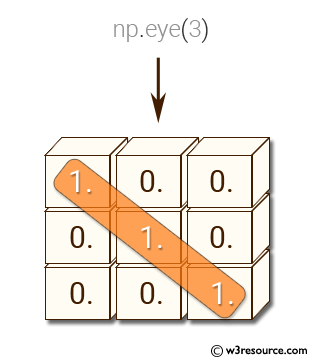﻿ NumPy: Create a 3x3 identity matrix, i.e. diagonal elements are 1,the rest are 0 - w3resource# NumPy: Create a 3x3 identity matrix, i.e. diagonal elements are 1,the rest are 0

## NumPy: Basic Exercise-27 with Solution

Write a NumPy program to create a 3x3 identity matrix, i.e. diagonal elements are 1,the rest are 0.

Sample Solution :

Python Code :

``````import numpy as np
x = np.eye(3)
print(x)
``````

Sample Output:

```[[ 1.  0.  0.]
[ 0.  1.  0.]
[ 0.  0.  1.]]
```

Pictorial Presentation:Python Code Editor:

Have another way to solve this solution? Contribute your code (and comments) through Disqus.

What is the difficulty level of this exercise?

Test your Python skills with w3resource's quiz

﻿

## Python: Tips of the Day

Checks if the given number falls within the given range.

Example:

```def tips_range(n, start, end = 0):
return start <= n <= end if end >= start else end <= n <= start
print(tips_range(2, 4, 6))
print(tips_range(4, 8))
print(tips_range(1, 3, 5))
print(tips_range(1, 3))
```

Output:

```False
True
False
True
```